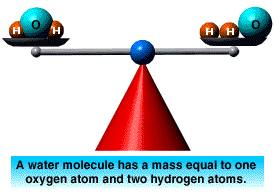## Illustrate law of conservation of mass, Chemistry

Assignment Help:

Q. Illustrate Law of Conservation of Mass?

Ans:

Conservation of Mass and Atoms:

The Law of Conservation of Mass states that mass is neither created nor destroyed. Therefore, the total mass of matter before and after a chemical change remains the same. The number of atoms also remains the same. The only thing that changes is the arrangement of the atoms.

For example, the sum of the masses of hydrogen and oxygen before they combine to form water is the same as the total mass of the water they form. Furthermore, the sum of the number of atoms of hydrogen and oxygen is the same as the total number of atoms in the water.The only change that occurs in a chemical reaction is in the arrangement of the atoms. Prior to the reaction, the hydrogen and oxygen atoms are not bonded to one another, and therefore exist separately. However, after the reaction, the hydrogen and oxygen atoms are bonded to one another.

#### STATES OF MATTER, USE THE KINETIC PARTICLE THEORY TO EXPLAIN THE EXISTENCE ...

USE THE KINETIC PARTICLE THEORY TO EXPLAIN THE EXISTENCE OF THE DIFFERENT STATES OF MATTER

#### Number of unpaired electrons in a chromic ion, The number of unpaired elect...

The number of unpaired electrons in a chromic ion Cr 3+ (atomic number = 24) is: (1) 6        (2) 4        (3) 3        (4) 1 Ans: 3

Question .. #

#### What is the formula of the compound, A cubic solid is made of two elements ...

A cubic solid is made of two elements X and Y. Atoms V are at the corners of the cube and X at the body centre. What is the formula of the compound?

#### Ion mobility, Ion mobility: Ion mobility is related to molar conducti...

Ion mobility: Ion mobility is related to molar conductivity by the equations: which allows the mobilities, u+ and u- of the cation and anion to be determined from mol

#### #alkyenes, reactions for preparation of westron

reactions for preparation of westron

#### Hardness of water, Why does calcium sulphate form scales in high pressure b...

Why does calcium sulphate form scales in high pressure boilers

#### Solution, How we get 49 gm = 1 eqv

How we get 49 gm = 1 eqv

#### Why formal charge does not give an accurate picture, Why Formal charge does...

Why Formal charge does not give an accurate picture Formal charge does not give an accurate picture, due to O is more electronegative than H; most of the positive charge is rea